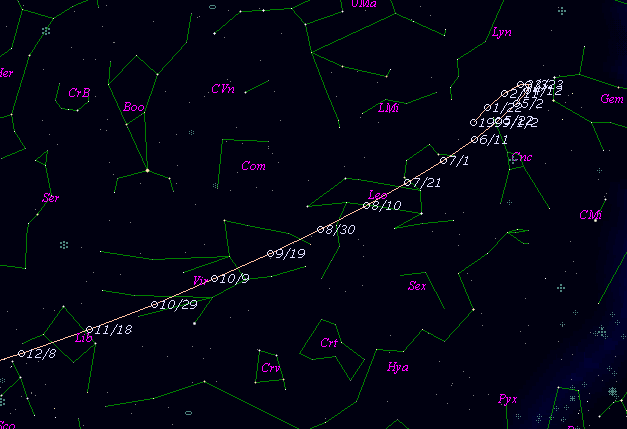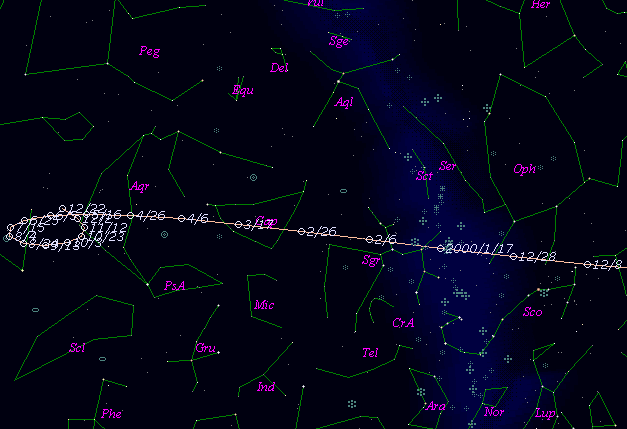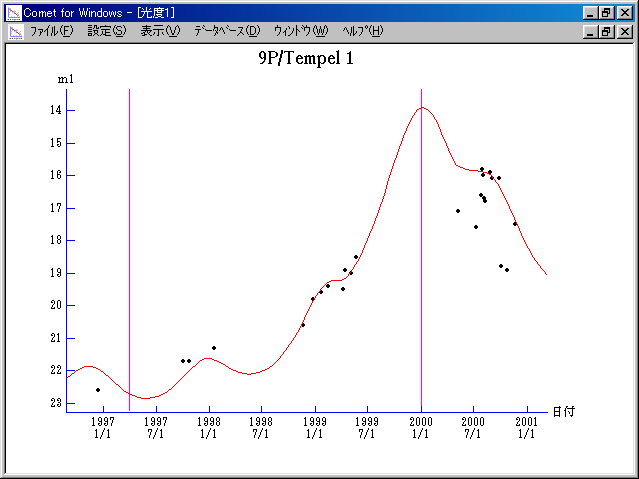# 9P/Tempel 1 (2000)###Orbital Elements

```   The following improved orbital elements by Kenji Muraoka,
are from 461 observations 1967 to 1998, including 9 Planets,
Moon and 5 minor planets perturbations and non-gravitational
effect of style II.  The mean residual is +/- 0.90 arc seconds.

Epoch  =  2000 Jan. 17.0  TT       JDT = 2451560.5
T  =  2000 Jan.  2.61656       +/- 0.00023 (m.e.) TT
Peri. =  178.91098                +/- 0.00012
Node  =   68.96653                +/- 0.00007   (2000.0)
Incl. =   10.54133                +/- 0.00001
q  =    1.5000475              +/- 0.0000004 AU
e  =    0.5189535              +/- 0.0000001
a  =    3.1183002              +/- 0.0000004 AU
n  =    0.17898935             +/- 0.00000003
P  =    5.507                  +/- 0.0000010  years
A1  =   +0.013                  +/- 0.004
A2  =   +0.00178                +/- 0.00002
```

###Finding Charts###Magnitudes Graph

```        m1 = 14.0 + 5 log d +  7.5 log r  [    ,-270]  (             - 1999 Apr.  7)
m1 =  8.5 + 5 log d + 20.0 log r  [-270, 120]  (1999 Apr.  7 - 2000 May   1)
m1 = 12.1 + 5 log d +  6.7 log r  [ 120,    ]  (2000 May   1 -             )
```##### The orbital elements are calculated by Kenji Muraoka. The charts are made with StellaNavigator Ver.2.0 for Windows (AstroArts / ASCII). The magnitudes graphs are made with Comet for Windows.